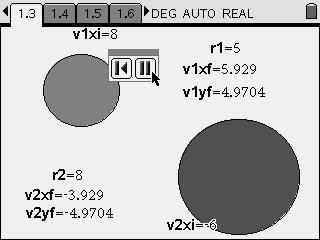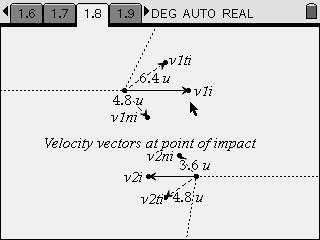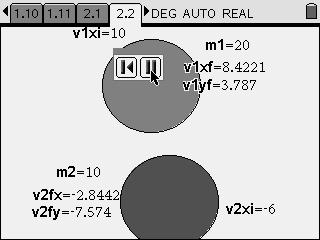# Activities

••• ##### Subject Area

• Science: Physics: Work, Energy and Momentum

• ##### Author9-12

45 Minutes

• ##### Device
• TI-Nspire™
• TI-Nspire™ CAS
• ##### Software

TI-Nspire™
TI-Nspire™ CAS

1.7

• ##### Report an Issue

Two-Dimensional Collisions#### Activity Overview

Students observe animations of two-dimensional elastic collisions of spheres and then calculate linear momenta and kinetic energies.

#### Key Steps

•Students investigate the relationships between velocity, mass, and momentum during elastic collisions.

First, students examine a simulation of the collision of two objects of equal mass. Students can vary the radius and initial velocity of each object. They then observe an animation showing the collision of the objects. They explore the conditions necessary for collision to occur.

•Then, students examine a diagram showing the velocity vectors of the objects at the moment they collide. They measure the lengths of the objects' velocities parallel and perpendicular to a line connecting the centers of the two objects.

•Finally, students explore an animation of two objects of equal radius colliding. They vary the masses of the objects and observe the resulting velocity vectors. They use these observations to show that both linear momentum and kinetic energy are conserved during perfectly elastic collisions of objects of unequal mass and unequal velocity.